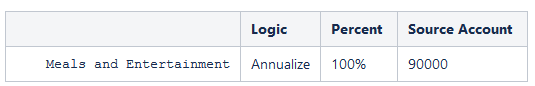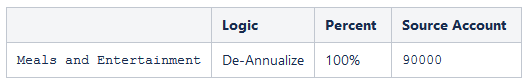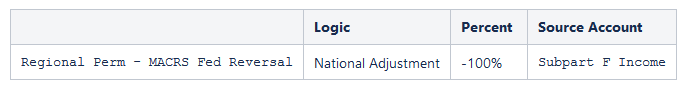## Tax Automation Logic Examples

When you set up Tax Automation, you can select the logic to be applied to the account. Logic selections include Pull, Movement, Squeeze, Annualize, DeAnnualize, National Adjustment, and Prior Year.

The following sections are examples of Annualize, DeAnnualize, National Adjustment, and Prior Year logic.

For steps on running Tax Automation, see Working with National Tax Automation and Working with Regional Tax Automation.

Annualize—Allows partial year amounts to be annualized to a full year amount.

Example:

The trial balance has been loaded in P03, and the Meals and Entertainment expense for the three months ending March 31 is \$36,000. To annualize to a full year, or 12-month estimate, the \$36,000 amount must be converted to a monthly amount or 12,000, and then converted to a full 12 month amount or 144,000, as follows:If the above rule is set for Period P1 then:

`Assuming value at Source Account (90000) is \$12,000`

`Monthly periods (\$12,000/1) * 12 = \$144,000`

If the above rule is set for Period P3 then:

`Assuming value at Source Account (90000) is 12000`

`Monthly periods (\$12,000/3) * 12 = \$48,000`

If the above rule is set for Period P6 then:

`Assuming value at Source Account (90000) is 12000`

`Monthly periods (\$12,000/6) * 12 = \$24,000`

If the above rule is set for Period P12 then:

`Assuming value at Source Account (90000) is 12000`

`Monthly periods (\$12,000/12) * 12 = \$12,000`

DeAnnualize—Allows full year values to be De-Annualized to a partial year amount based on the period of the rule.

Example:

The Meals and Entertainment expense for the prior year (12 months) ending Dec 31st 2019 is \$144,000. To de-annualize to a partial year, or 3-month estimate, the \$144,000 amount must be converted to a monthly amount or 12,000, and then converted to a 3 month value or 36,000, as follows:If the above rule is set for Period P1 then:

`Assuming value at Source Account (Meals and Entertainment) is 12,000`

`Monthly periods (\$144,000/12) * 1(Period) = \$12,000`

If the above rule is set for Period P3 then:

`Assuming value at Source Account (90000) is 12000`

`Monthly periods (\$144,000/12) * 3 = \$36,000`

If the above rule is set for Period P6 then:

`Assuming value at Source Account (90000) is 12000`

`Monthly periods (\$144,000/12) * 6 = \$72,000`

If the above rule is set for Period P9 then:

`Assuming value at Source Account (90000) is 12000`

`Monthly periods (\$144,000/12) * 9 = \$108,000`

If the above rule is set for Period P12 then:

`Assuming value at Source Account (90000) is 12000`

`Monthly periods (\$144,000/12) * 12 = \$144,000`

In case of Pull and Annualize, it will be accumulated.

National Adjustment—Allows for adjustment (for example, reversal) of a National Permanent or Temporary Difference in the Regional Provision.

Example:

There is a Permanent Difference in the Current Provision for Subpart F income in the amount of \$100,000. However, the state of Illinois does not tax Subpart F income and therefore is required to reverse this adjustment in a state Permanent Difference account. To reverse the amount, you would apply National Adjustment logic with a percentage of minus 100%.

`Any period \$100,000 * -100% = \$-100,000`

Valid Setting - This logic is valid for Tax accounts. Source Account for National Adjustment logic defaults to the Target account. Source RollForward is disabled for National Adjustment logic.If the above rule is set for Period P12 then:

`Assuming value at Source Account (Subpart F Income) is 100,000`

`Regional Perm - MACRS Fed Reversal = -\$100,000`

Prior Year—Provides the ability to bring the prior year data into a provision.

Example:

There is a Permanent Difference in the Current Provision for tax exempt interest in the amount of \$-1,000,000 in the prior year Actual Scenario. You want to use the prior year amount of the tax exempt interest as an estimate in the current year Forecast Scenario. To use the amount, you would apply Prior Year logic with a percentage of 100%.

`Any period -\$1,000,000 * 100% = -1,000,000`

Default Setting - The default for the Year is the current year minus 1. The default for the Period is P12.

Note:

Target account is the Source account. When using the Prior Year method, you must specify the Source Movement. it applies only to Automated movement.

Default source Scenario is the target Scenario.

Form Validations

The system performs the following validations for the Tax automation forms (Global / Domicile / Entity / Entity Regional) when you use Prior Year or National Adjustment logic. If you select an invalid account or movement, the system displays an error message.

Table 22-2 Form Validations for Prior Year and National Adjustment

Logic Target Account Valid/Invalid Selection Source Account Valid/Invalid Selection Target Movement Valid Selection Source Movement Valid Selection
Prior Year   Must be same as target tax Account.

Target movement must be <alias> (TRCS_CySYS).

TRCS_CurrentTaxCYTotal (Default) (No Selection allowed).

Source movement must be <alias> (TRCS_CurrentTaxCYTotal).

National Adjustment Target Account must be Regional Perm / Temp Account. Source Account must have NationalAndRegional as TaxDataType property.

National Adjustment rule is only valid to be set on regional tax accounts.

Target movement must be <alias> (TRCS_CySYS).

TRCS_CurrentTaxCYTotal (Default) (No Selection allowed).

Source movement must be <alias> (TRCS_CurrentTaxCYTotal).